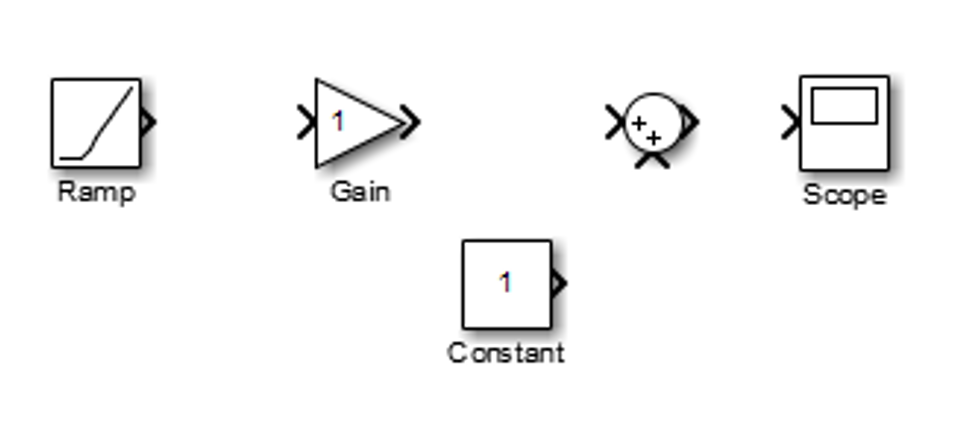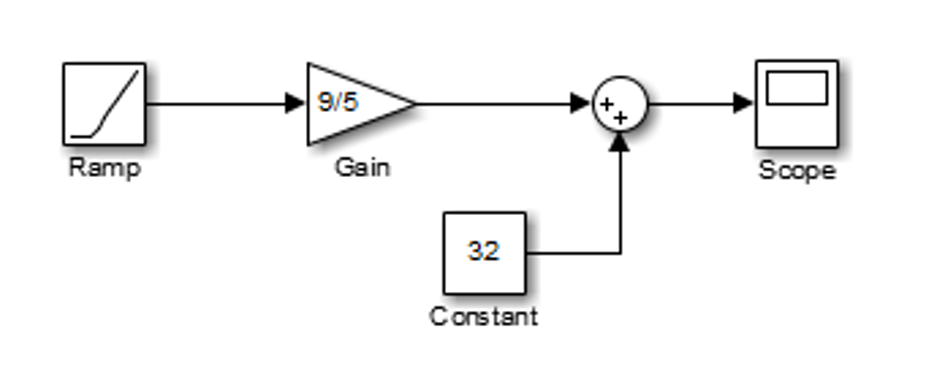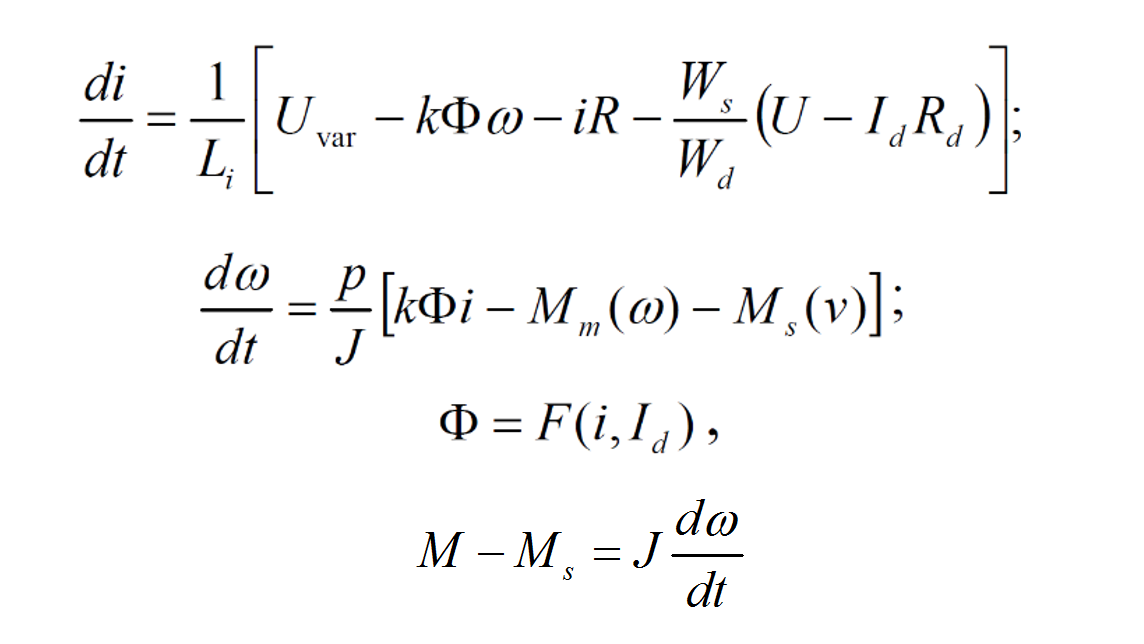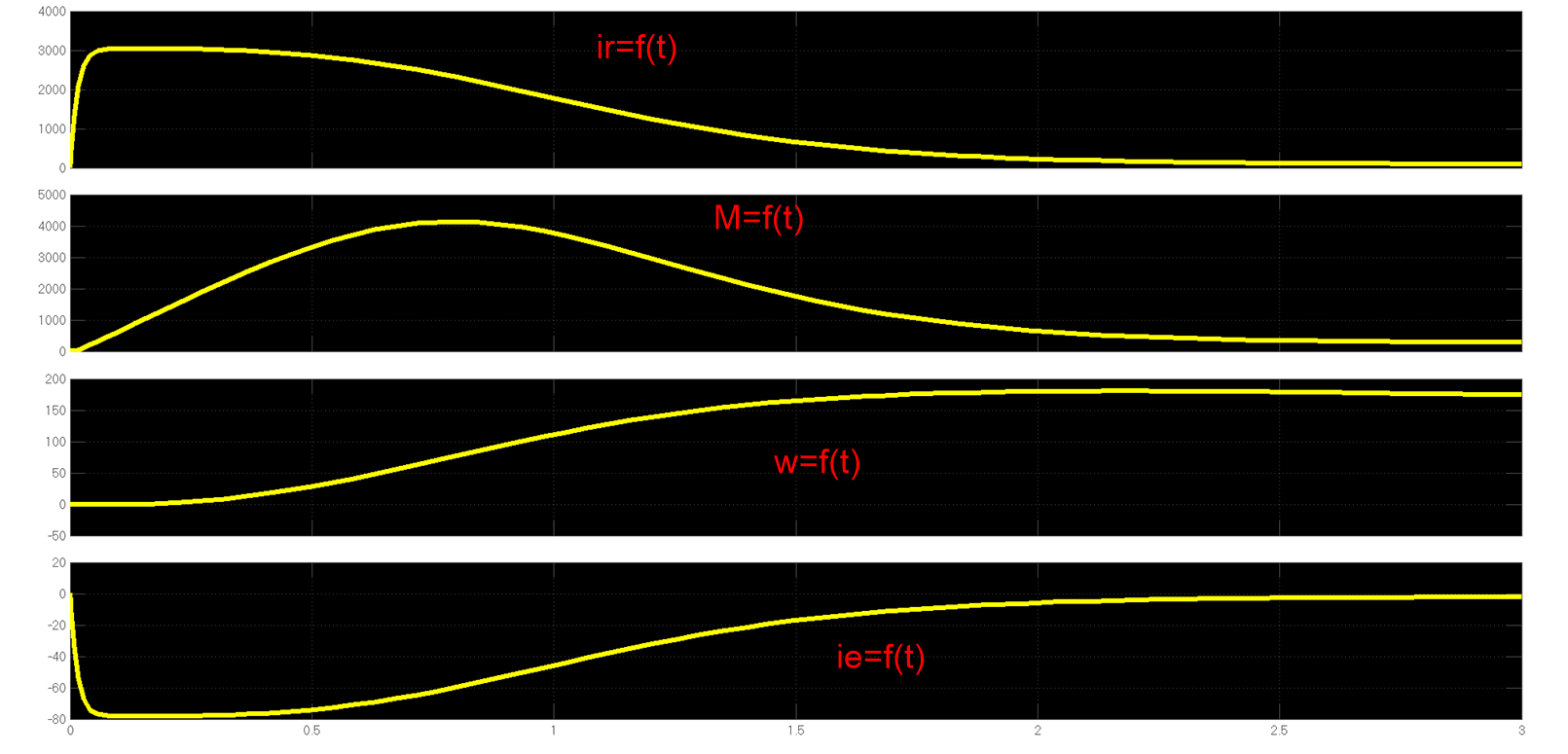# Mathematical modelling with MATLAB Simulink

December 23, 20160Mathematical model – a representation in mathematical terms of the behavior of real devices and objects. In the practices of science and engineering design, models are often applied to predict what will happen in a future situation.

In engineering design, however, the predictions are used in ways that have far different consequences than simply anticipating the outcome of an experiment. Every new building or airplane, for example, represents a model-based prediction that the building will stand or the airplane will fly without dire, unanticipated consequences. Thus, beyond simply validating a model, prediction in engineering design assumes that resources of time, imagination and money can be invested with confidence because the predicted outcome will be a good one.

Mathematical models are critical to understanding and accurately predicting the behavior of complex systems. These models enable critical tasks, such as:

• Forecasting and optimizing system behavior;
• Designing control systems;
• Characterizing system response.

MathWorks products provide all the tools you need to develop mathematical models. MATLAB supports both numeric and symbolic modeling approaches and provides curve fitting, statistics, optimization, ODE and PDE solving, calculus, and other core mathematical tools. Simulink adds an environment for modeling and simulating the behavior of multi domain systems and for developing embedded systems.

#### How to model a simple equation

Linearity is one of the most important concepts in mathematical modeling. Models of devices or systems are said to be linear when their basic equations are such that the magnitude of their behavior or response produced is directly proportional to the excitation or input that drives them.

Here is the equation that converts Celsius temperature to Fahrenheit: TF = 9/5(TC) + 32

First, consider the blocks needed to build the model:

• A Ramp block to input the temperature (TC) signal, from the Sources library. The Ramp block generates a signal that starts at a specified time and value and changes by a specified rate;
• A Constant block to define a constant of 32, also from the Sources library;
• A Gain block to multiply the input signal by 9/5, from the Math Operations library. This block multiplies the input by a constant value (gain). The input and the gain can each be a scalar, vector, or matrix;
• A Sum block to add the two quantities, also from the Math Operations library. It performs the addition or subtraction on its inputs (scalar, vector, or matrix). This block can also collapse the elements of a signal;
• A Scope block to display the output, from the Sinks library. It provides several methods for displaying the simulated data and capturing the output for the later analysis.
•   Next, let’s gather the blocks into our model window:Now assign the parameter values to the Gain and Constant blocks by opening (double-clicking) each block and entering the appropriate values. Then, click the OK button to apply the value and close the dialog box. Connect the blocks.The Ramp block inputs the Celsius temperature. Open that block and set the initial output parameter to 0. The Gain block multiplies that temperature by the constant 9/5. The Sum block adds the value 32 to the result and outputs the Fahrenheit temperature.

#### Mathematical model of a Complex System

As an example for mathematical modelling of a complex system can serve the DC compound motor. The complexity of this system is due to some differential equations that describe the motor’s functionality. A system of ordinary differential equations has the following characteristics:

• All of the equations are ordinary differential equations;
• Each equation is the derivative of a dependent variable with respect to one independent variable, usually time;
• The number of equations is equal to the number of dependent variables in the system.

Here are the equations of the mathematical model of the DC motor:Where:

• U, Uvar – contact network tension and respectively Chopper’s exit tension;
• Id, i – the shunt current and respectively the armature current;
• Wd, Ws – turns number of the shunt and series coils;
• Li – armature and auxiliary poles inductivity;
• Ф – useful magnetic flux;
• w –rotation speed;
• Mm(&)=Pmec/& – the torque of mechanical losses Pmec as rotor speed function;
• Ms(v) – load torque as a function of trolleybus speed v;
• J – the total moment of inertia.

The Simulink model of the motor was validated proceeding from experimental tests. In laboratory conditions the DK 210A was tested with the following datum:

• Nominal power: Pn=110 kW
• Armature voltage: Un=550 V
• Number of poles: p=2
• Rotor resistance: Rr=0.181 Ohm
• Rotor inductility: Lr=0.0026 Hn
• Number of rotor wound : Ws=24
• Stator resistance: Rd=125 Ohm
• Number of excitation wounds Wd=930
• Moment of inertia J=41.66 kg m2
Fig. 1. DC compounded magnetization curves

Using this information it is possible to create the MATLAB Simulink model of the DC motor:

Fig.2. MATLAB Simulink model of the DC motor with compounded excitation

Initial values of this model are: armature voltage (Ur), excitation voltage (Ue) and load torque (Ms). So it is possible to create characteristics of armature current (ir), motor torque (M), motor speed (w) and excitation current (ie).

Fig.3. Block scheme of the DC modelFig.4. The characteristics of the DC motor

#### Conclusions

Simulation software is becoming more and more important in the electrical engineering education due to some advantages against the hands-on models, which are very useful due to the similitude with the real industry installation. In case the measurement blocks on the diagram become numerous, MATLAB Simulink provides a list of results which can be exported to multiple file formats, as example – the xls spreadsheet.

Mathematical model of a DC motor helps us to test its behavior without using complicated equipment that usually costs a lot of money and requires much more time to install and configure it.

The most important advantage of Simulink is its flexibility, it can simulate complex systems only by knowing the required equations and it can work with continuous or discrete values. The system can be created using physical or mathematical blocks. MATLAB Simulink has a large library with examples of models from different engineering fields.##### Connect with us
Bulgara Street 33/1, Chisinau MD-2001, Moldova
+ 373 22 996 170
info@isd-soft.com
De Amfoor 15, 5807 GW Venray-Oostrum, The Netherlands
+ 31 478 502944